# One-sample t-test using Minitab

## Introduction

The one-sample t-test is used to determine whether a sample comes from a population with a specific mean. This population mean is not always known, but is sometimes hypothesized.

For example, imagine that an academic was conducting research on the relationship between exam performance and revision time, but wanted to first check whether his 100 participants reflected the national average in terms of their academic ability, measured in terms of their GMAT score. The academic could use a one-sample t-test to compare the GMAT score of the 100 participants against the national average. Alternately, imagine that a lecturer believed her course required 10 hours of study time per week and wanted to determine whether students spent this amount of time studying. The lecturer could use a one-sample t-test to compare the weekly study time of a sample of 20 students to the suggested 10 hours.

In this guide, we show you how to carry out a one-sample t-test using Minitab, as well as interpret and report the results from this test. However, before we introduce you to this procedure, you need to understand the different assumptions that your data must meet in order for a one-sample t-test to give you a valid result. We discuss these assumptions next.

## Assumptions

A one-sample t-test has four assumptions. You cannot test the first two of these assumptions with Minitab because they relate to your study design and choice of variables. However, you should check whether your study meets these two assumptions before moving on. If these assumptions are not met, there is likely to be a different statistical test that you can use instead. Assumptions #1 and #2 are explained below:

• Assumption #1: Your dependent variable should be measured at a continuous level (i.e., it is an interval or ratio variable). Examples of continuous variables include height (measured in feet and inches), temperature (measured in °C), salary (measured in US dollars), revision time (measured in hours), intelligence (measured using IQ score), firm size (measured in terms of the number of employees), age (measured in years), reaction time (measured in milliseconds), grip strength (measured in kg), power output (measured in watts), test performance (measured from 0 to 100), sales (measured in number of transactions per month), academic achievement (measured in terms of GMAT score), and so forth. If you are unsure whether your dependent variable is continuous (i.e., measured at the interval or ratio level), see our Types of Variable guide.
• Assumption #2: The data are independent (i.e., not correlated/related), which means that there is no relationship between the observations. This is more of a study design issue than something you can test for, but it is an important assumption of the one-sample t-test.

Assumptions #3 and #4 relate to the nature of your data and can be checked using Minitab. You have to check that your data meets these assumptions because if it does not, the results you get when running a one-sample t-test might not be valid. In fact, do not be surprised if your data violates one or more of these assumptions. This is not uncommon. However, there are possible solutions to correct such violations (e.g., transforming your data) such that you can still use a one-sample t-test. Assumptions #3 and #4 are explained below:

• Assumption #3: There should be no significant outliers. An outlier is simply a case within your data set that does not follow the usual pattern. For example, consider a study examining the test anxiety of 500 students where anxiety was measured on a scale of 0-100, with 0 = no anxiety and 100 = maximum anxiety. The mean test anxiety score was 56 and the vast majority of students scored between 42 and 70. However, one student scores just 2 on the scale, with the second lowest test anxiety score being 36. As such, a student scoring just 2 on the scale "could" be considered an outlier. Where a score is an outlier this is problematic because outliers can have a disproportionately negative effect on the one-sample t-test, reducing the accuracy of its results. Fortunately, when using Minitab to run a one-sample t-test on your data, you can easily detect possible outliers.
• Assumption #4: Your dependent variable should be approximately normally distributed. We talk about the one-sample t-test only requiring approximately normal data because it is quite "robust" to violations of normality, meaning that the assumption can be a little violated and still provide valid results. You can test for normality using the Shapiro-Wilk test of normality, which is easily tested for using Minitab.

In practice, checking for assumptions #3 and #4 will probably take up most of your time when carrying out a one-sample t-test. However, it is not a difficult task, and Minitab provides all the tools you need to do this.

In the section, Test Procedure in Minitab, we illustrate the Minitab procedure required to perform a one-sample t-test assuming that no assumptions have been violated. First, we set out the example we use to explain the one-sample t-test procedure in Minitab.

## Example

A researcher plans to conduct a study to determine how three different types of treatment affect depression. However, before the researcher carries out this study, he wants to make sure that the 40 participants taking part have depression scores that are considered to be 'normal'. Let's imagine that a score of 4.0 is considered to reflect 'normal' depression. Lower scores indicate less depression and higher scores indicate greater depression. Therefore, the depression of all 40 participants is measured and a one-sample t-test is used to determine whether this sample is representative of a normal population (i.e., whether the sample-estimated population mean score is significantly different from 4.0). The depression scores are recorded in the variable, Depression score.

## Setup in Minitab

In Minitab, we set up the dependent variable, Depression score, under column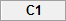. Then, we entered the scores on the dependent variable (i.e., the depression score for each participant) into the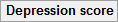column. This is illustrated below: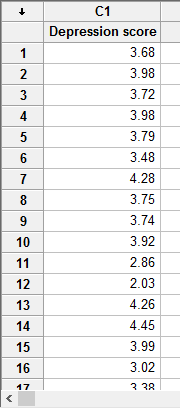Published with written permission from Minitab Inc.

Note: If you do not have all the data for your dependent variable, unlike our example above, but only the summarized data (i.e., the sample size, mean and standard deviation), you will need to set up your data differently.

## Test Procedure in Minitab

In this section, we show you how to analyze your data using a one-sample t-test in Minitab when the four assumptions in the previous section, Assumptions, have not been violated. Therefore, the three steps required to run a one-sample t-test in Minitab are shown below:

• Click Stat > Basic Statistics > 1-Sample t... on the top menu, as shown below: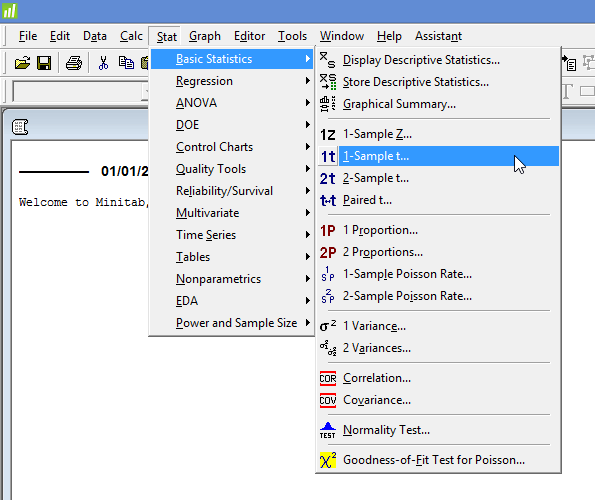Published with written permission from Minitab Inc.

You will be presented with the following 1-Sample t (Test and Confidence Interval) dialogue box: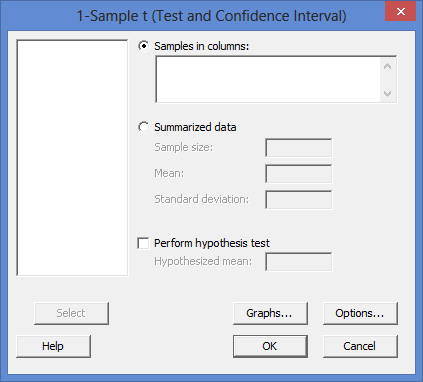Published with written permission from Minitab Inc.

• Leave the Samples in columns option selected and enter the dependent variable, Depression score, into the box underneath. You will end up with the dialogue box shown below: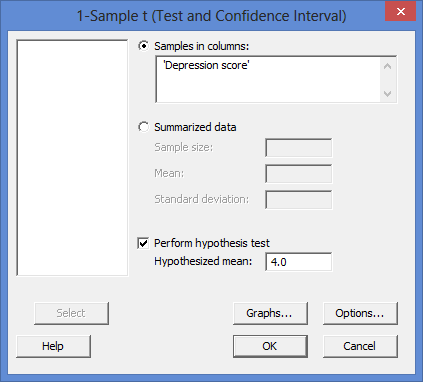Published with written permission from Minitab Inc.

Explanation: To transfer the dependent variable, Depression score, you first need to click inside the Samples in columns: box for the dependent variable to appear in the main left-hand box (e.g., C1   Depression score). This will activate the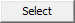button (it is usually faded: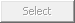). Next, select C1   Depression score and then either press thebutton or simply double-click on C1   Depression score.

Note 1: By default, Minitab uses 95% confidence intervals, which equates to declaring statistical significance at the p < .05 level. If you want to change this, you can do so by first clicking on the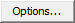button, which opens the 1-Sample t - Options dialogue box, as shown below: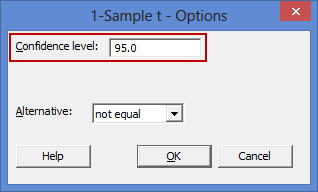To change the value of the confidence interval, simply click into the Confidence level: box – highlighted in red above – and change the value (e.g., a vale of 99.0 would equate to declaring statistical significance at the p < .01 level).

Note 2: In addition to the procedure above, there is another method for carrying out a one-sample t-test in Minitab (highlighted in the red rectangle below).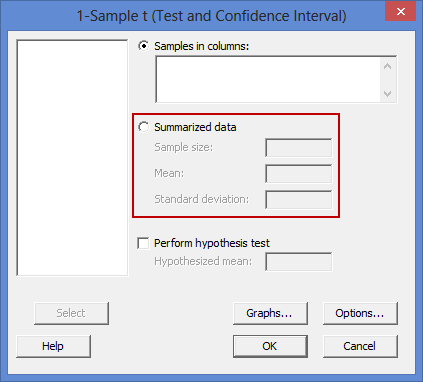This alternative method is useful if you only have the summarized data (e.g., the sample size, mean and standard deviation for your dependent variable), rather than all the scores.

## Output of the one-sample t-test in Minitab

The Minitab output for the one-sample t-test is shown below: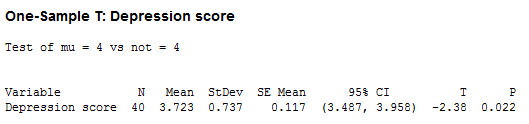You will be presented with descriptive statistics including the sample size (the "N" column), mean (the "Mean" column), standard deviation (the "StDev" column) and the standard error of the mean ("SE Mean" column), as well as the 95% confidence interval (CI) of the mean ("95% CI"). Finally, the results of the one-sample t-test include the value of the known or hypothesized population mean you are comparing your sample data to (the Test of mu = 4 vs not = 4 row), the observed t-value (the "T" column) and the statistical significance (2-tailed p-value) of the one-sample t-test (the "P" column).

In our example, you can see that the 'normal' depression score value was "4" (the Test of mu = 4 vs not = 4 row). The sample size (N) was 40, the mean depression score (Mean) was 3.723, with a standard deviation of 0.737, and the 95% confidence interval of the mean was 3.487 to 3.958. The observed t-value (T) was -2.38 and the statistical significance was .022. The degrees of freedom were 39, but this is not shown in the Minitab output (N.B., this is simply the sample size, N, minus 1; so 40 – 1, which is 39). Therefore, it can be concluded that the population means are statistically significantly different.

Note: We present the output from the one-sample t-test above. However, since you should have tested your data for the assumptions we explained earlier in the Assumptions section, you will also need to interpret the Minitab output that was produced when you tested for them. This includes: (a) the boxplots you used to check if there were any significant outliers; and (b) the output Minitab produces for your Shapiro-Wilk test of normality to determine normality. Also, remember that if your data failed any of these assumptions, the output that you get from the one-sample t-test procedure (i.e., the output we discuss above) might no longer be valid, but you may be able to use another statistical test instead.

## Reporting the output of the one-sample t-test

When you report the output of your one-sample t-test, it is good practice to include:

• A. An introduction to the analysis you carried out.
• B. Information about your sample (N).
• C. A statement of whether the one-sample t-test was statistically significant, including the mean (Mean) and standard deviation (StDev), 95% confidence interval of the mean (95% CI), t-value (T), degrees of freedom, and significance level, or more specifically, the 2-tailed p-value (P).

Based on the Minitab output above, we could report the results of this study as follows:

• General

A one-sample t-test was run to determine whether participants' depression score was different to normal, defined as a depression score of 4.0. Mean depression score (3.72, 95% CI, 3.49 to 3.96) was lower than the normal depression score of 4.0, a statistically significant difference, t(39) = -2.83, p = .022.

Portions of information contained in this publication/book are printed with permission of Minitab Inc. All such material remains the exclusive property and copyright of Minitab Inc. All rights reserved.